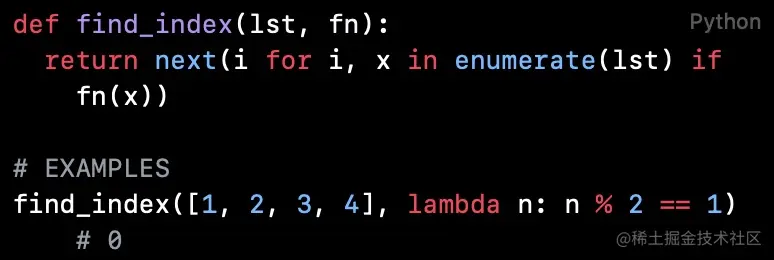# Python code reading (Part 44): find the location of qualified elements

2022-01-30 08:09:10 FelixZPython Code reading collection Introduction ： Why not recommend Python Beginners directly look at the project source code

The code read in this article implements the search for the location of qualified elements in the list .

## `find_index`

``````def find_index(lst, fn):
return next(i for i, x in enumerate(lst) if fn(x))

# EXAMPLES
find_index([1, 2, 3, 4], lambda n: n % 2 == 1) # 0
Copy code ``````

`find_index` Function receives a list and a discriminant function , Returns the subscript of the first element that makes the discriminant function true .

The function uses a list derivation 、`enumerate()` and `next()` Come back to `lst` First of all Is a discriminant function `fn` The return of is `True` The index of the element .

`next(iterator[, default])` Get the next element of the iterator . If the iterator runs out , Returns the given default, If there is no default, trigger `StopIteration`

`enumerate(iterable, start=0)` Returns an enumeration object .`enumerate()` Of the iterator returned `__next__()` Method returns a tuple , It contains a count value （ from `start` Start , The default is `0`） And through iteration `iterable` Value gained . If `start` from `0` Start , Equivalent to getting the subscript of the list .

``````>>> seasons = ['Spring', 'Summer', 'Fall', 'Winter']
>>> list(enumerate(seasons))
[(0, 'Spring'), (1, 'Summer'), (2, 'Fall'), (3, 'Winter')]
>>> list(enumerate(seasons, start=1))
[(1, 'Spring'), (2, 'Summer'), (3, 'Fall'), (4, 'Winter')]
Copy code ``````

## `find_last_index`

Allied , Can achieve `find_last_index` function , Use `lst[::-1]` Slice form , Reverse the list . And then use `len(lst)` And the position of the first element in the inverted list that conforms to the discriminant function , Calculate the position of the element in the original list .

``````def find_last_index(lst, fn):
return len(lst) - 1 - next(i for i, x in enumerate(lst[::-1]) if fn(x))

# EXAMPLES
find_last_index([1, 2, 3, 4], lambda n: n % 2 == 1) # 2
Copy code ``````